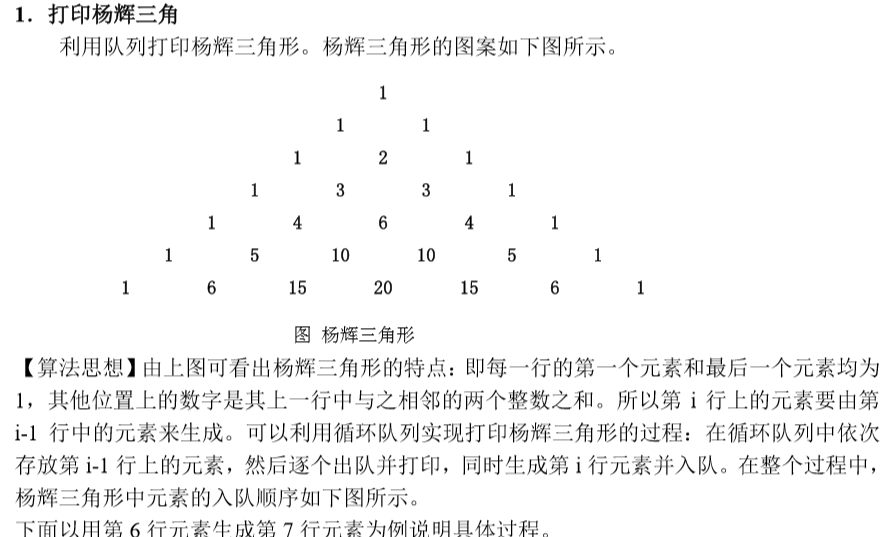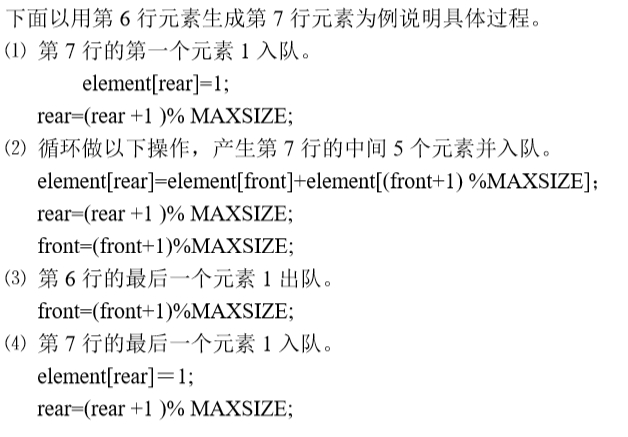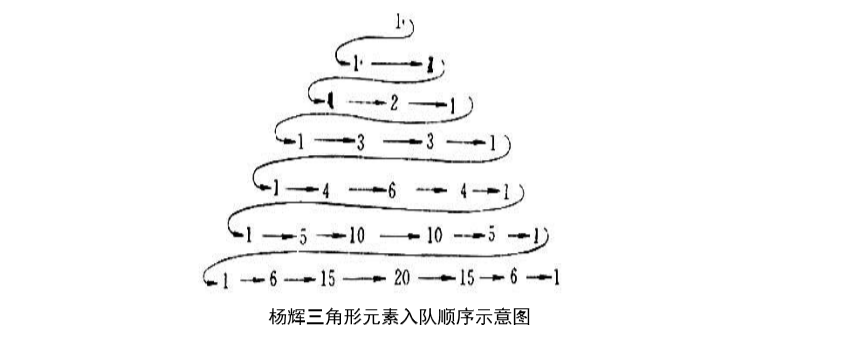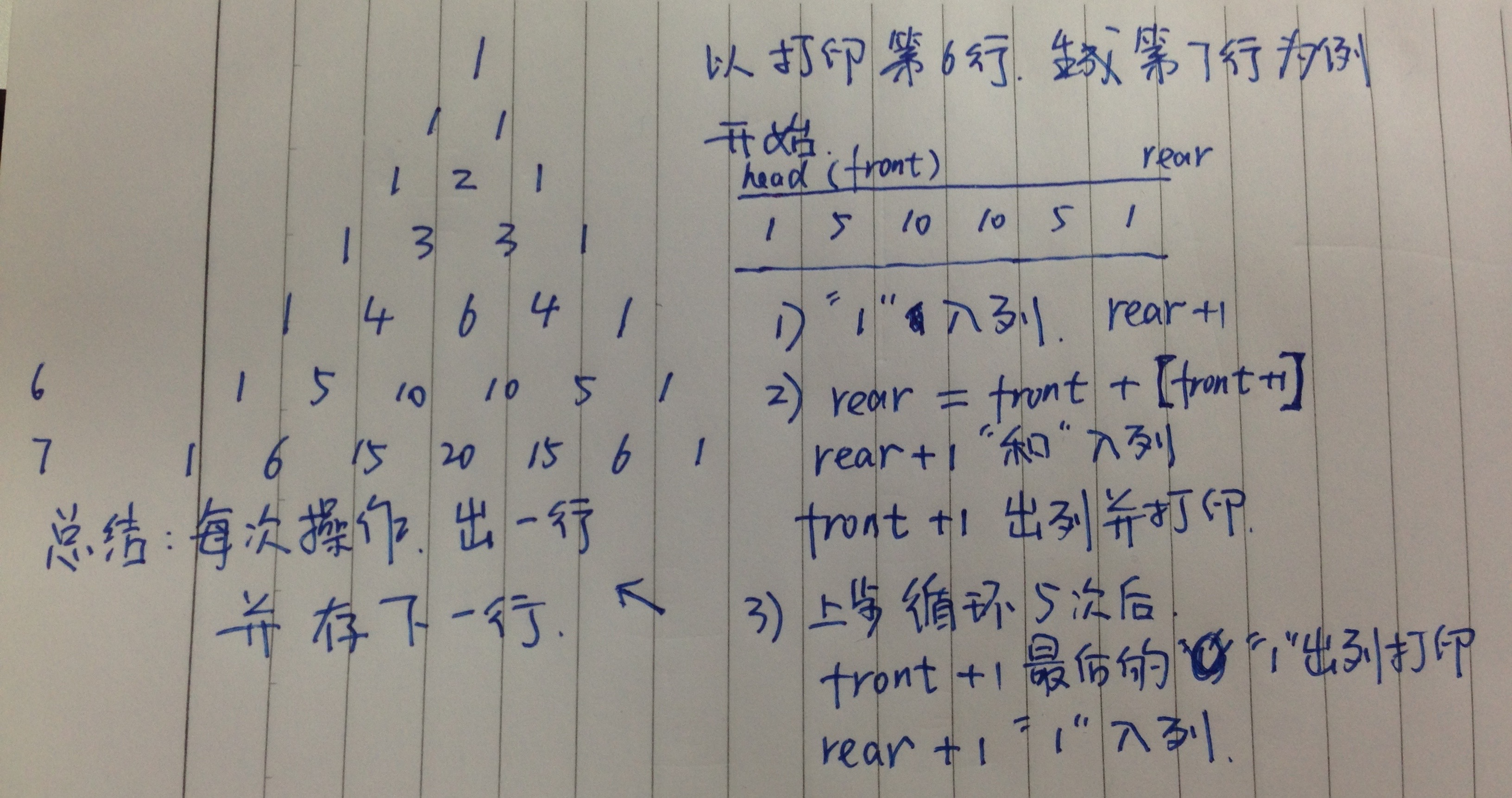• ## 队列的应用举例

千次阅读 2012-11-01 11:14:05
本算法要求找一条迷宫最短路径，算法基本思想为：从迷宫入口点（1,1）出发，向四周搜索，记下所有一步能到达坐标点；然后依次再从这些点出发，再记下所有一步能到达坐标点，…，依此类推，直到到达迷宫...
例3.5 求迷宫的最短路径：http://see.xidian.edu.cn/cpp/html/959.html
现要求设计一个算法找一条从迷宫入口到出口的最短路径。本算法要求找一条迷宫的最短路径，算法的基本思想为：从迷宫入口点（1,1）出发，向四周搜索，记下所有一步能到达的坐标点；然后依次再从这些点出发，再记下所有一步能到达的坐标点，…，依此类推，直到到达迷宫的出口点(m,n)为止，然后从出口点沿搜索路径回溯直至入口。这样就找到了一条迷宫的最短路径，否则迷宫无路径。
有关迷宫的数据结构、试探方向、如何防止重复到达某点以避免发生死循环的问题与例3.2 处理相同，不同的是：如何存储搜索路径。在搜索过程中必须记下每一个可到达的坐标点，以便从这些点出发继续向四周搜索。由于先到达的点先向下搜索，故引进一个“先进先出”数据结构------队列来保存已到达的坐标点。到达迷宫的出口点(m,n)后，为了能够从出口点沿搜索路径回溯直至入口，对于每一点，记下坐标点的同时，还要记下到达该点的前驱点，因此，用一个结构数组sq[num]作为队列的存储空间，因为迷宫中每个点至多被访问一次，所以num
至多等于m*n。sq 的每一个结构有三个域：x,y 和pre，其中x,y 分别为所到达的点的坐标，pre 为前驱点在sq 中的坐标，是一个静态链域。除sq 外，还有队头、队尾指针：front 和rear 用来指向队头和队尾元素。
队的定义如下：
typedef struct
{ int x,y;
int pre;
}sqtype;
sqtype sq[num];
int front,rear;
初始状态，队列中只有一个元素sq记录的是入口点的坐标（１,１），因为该点是出发点，因此没有前驱点，pre 域为－１，队头指针front 和队尾指针rear 均指向它，此后搜索时都是以front 所指点为搜索的出发点，当搜索到一个可到达点时，即将该点的坐标及front 所指点的位置入队，不但记下了到达点的坐标，还记下了它的前驱点。front 所指点的８个方向搜索完毕后，则出队，继续对下一点搜索。搜索过程中遇到出口点则成功，搜索结束，打印出迷宫最短路径，算法结束；或者当前队空即没有搜索点了，表明没有路径算法也结束。
算法如下：
void path(maze，move)
int maze[m][n]；/*迷宫数组*/
item move；/*坐标增量数组*/
{ sqtype sq[NUM];
int front,rear；
int x,y,i,j,v;
front=rear=0;
sq.x=1; sq.y=1; sq.pre=-1; /*入口点入队*/
maze[1,1]=-1;
while (front<=rear) /*队列不空*/
{ x=sq[front].x ; y=sq[front ].y ;
for (v=0;v<8;v++)
{ i=x+move[v].x; j=x+move[v].y;
if (maze[i][j]==0)
{ rear++;
sq[rear].x=i; sq[rear].y=j; sq[rear].pre=front;
maze[i][j]=-1;
}
if (i==m&&j==n)
{ printpath(sq,rear); /*打印迷宫*/
restore(maze); /*恢复迷宫*/
return 1;
}
} /*for v*/
front++; /*当前点搜索完，取下一个点搜索*/
} /*while*/
return 0;
} /*path*/
void printpath(sqtype sq[],int rear) /*打印迷宫路径*/
{ int i;
i=rear;
do { printf(＂ (%d,%d)

展开全文• 顺序循环队列的应用举例，约瑟夫环的实现，一共有n个人，从第m个开始报数，数到x的人出队，直到所有人出队
• 注意：所打印的杨辉三角形的最大行数一定要小于循环队列的 MAXSIZE 值。当然，本例用链队列也完全可以实现。 【算法描述】 void YangHuiTriangle() { SeqQueue Q; InitQueue(&Q); /* 初始化*/ EnterQueue(&...
 1．打印杨辉三角注意：所打印的杨辉三角形的最大行数一定要小于循环队列的 MAXSIZE 值。当然，本例用链队列也完全可以实现。
【算法描述】
void YangHuiTriangle()
{
SeqQueue Q;
InitQueue(&Q);           /* 初始化*/
EnterQueue(&Q, 1);       /* 第一行元素入队*/
for (n = 2; n <= N; n++) /* 产生第 n 行元素并入队，同时打印第 n-1 行的元素*/
{
EnterQueue(&Q, 1);           /* 第 n 行的第一个元素入队*/
for (i = 1; i <= n - 2; i++) /* 利用队中第 n-1 行元素产生第 n 行的中间 n-2 个元素 并入队*/
{
DeleteQueue(&Q, &temp);
Printf(“% d”, temp); /* 打印第 n-1 行的元素*/
GetHead(Q, &x);
temp = temp + x; /*利用队中第 n-1 行元素产生第 n 行元素*/
EnterQueue(&Q, temp);
}
DeleteQueue(&Q, &x);
printf(“% d”, x); /* 打印第 n-1 行的最后一个元素 */
EnterQueue(&Q, 1) /* 第 n 行的最后一个元素入队 */
}
while (!IsEmpty(Q)) /* 打印最后一行元素 */
{
DeleteQueue(&Q, &x);
printf(“% d”, x);
}
}

上面的算法只是逐个打印出了杨辉三角形前 N 层中的数据元素，并没有按三角形的形式输出，读者可以自己加入坐标数据，然后在屏幕上打印出杨辉三角形。
2．键盘输入循环缓冲区问题

在操作系统中，循环队列经常用于实时应用程序。例如，当程序正在执行其他任务时，用户 可以从键盘上不断键入所要输入的内容。系统在利用这种分时处理方法时，用户键入的内容 不能在屏幕上立刻显示出来，直到当前正在工作的那个进程结束为止。但在这个进程执行时， 系统是在不断地检查键盘状态，如果检测到用户键入了一个新的字符，就立刻把它存到系统 缓冲区中，然后继续运行原来的进程。当当前工作的进程结束后，系统就从缓冲区中取出键入的字符，并按要求进行处理。这里的键盘输入缓冲区采用了循环队列。队列的特性保证了 输入字符先键入、先保存、先处理的要求，循环结构又有效地限制了缓冲区的大小，并避免了假溢出问题。

【问题描述】
有两个进程同时存在于一个程序中。其中第一个进程在屏幕上连续显示字符 “A”，与此同时，程序不断检测键盘是否有输入，如果有的话，就读入用户键入的字符并 保存到输入缓冲区中。
在用户输入时，键入的字符并不立即回显在屏幕上。当用户键入一个 逗号（，）或分号(;)时，表示第一个进程结束，第二个进程从缓冲区中读取那些已键入的字符并显示在屏幕上。
第二个进程结束后，程序又进入第一个进程，重新显示字符“A”，同时用户又可以继续键入字符，直到用户输入一个分号（；）键，才结束第一个进程，同时也结束整个程序。
【算法描述】
#include <conio.h>
#include <queue.h>
#include <stdio.h>
main()
{ /*模拟键盘输入循环缓冲区*/
char ch1, ch2;
SeqQueue Q;
int f;
InitQueue(&Q); /* 队列初始化 */
for (;;)
{
for (;;) /*第一个进程*/
{
printf(“A”);
if (kbhit())
{
ch1 = getch(); /* 读取键入的字符，但屏幕上不显示 */
if (ch1 ==’;’|| ch1 ==’,’)
break; /* 第一个进程正常中断 */
f = EnterQueue(&Q, ch1);
if (f == FALSE)
{
printf(“循环队列已满\n”);
break; /* 循环队列满时，强制中断第一个进程*/
}
}
}
while (!IsEmpty(Q)) /*第二个进程*/
{
DeleteQueue(&Q, &ch2);
putchar(ch2); /*显示输入缓冲区的内容*/
}
if (ch1 ==’.’)
break; /*整个程序结束*/
}
}



展开全文• 队列的应用举例1（共2例） 打印杨辉三角  1  1 1  1 2 1  1 3 3 1  1 4 6 4 1  1 5 10 10 5 1  1 6 15 20 15 6 1 由上，是7行的杨辉三角形，杨辉三角形的特
队列的应用举例1（共2例）

打印杨辉三角

1
1  1
1  2  1
1  3  3  1
1  4  6  4  1
1  5  10 10 5  1
1  6  15 20 15 6  1

由上，是7行的杨辉三角形，杨辉三角形的特点是两腰都是数字1，其他位置是其上一行中与之相岭的两个整数之和。所以，在打印过程中，第i行上的元素要由第i－1行中的元素来生成。我们可以利用循环队列实现打印杨辉三角形的过程。在循环队列中依次存放第i－1行上的元素，然后逐个出列并打印，同时生成第i行元素并入列。
以打印第六行，生成第七行为例，如图前N行的算法描述如下：

void YangHuiTriangle(){
SeqQueue Q;
InitQueue(&Q);
EnterQueue(&Q,1); //第一行入列
for(n=2;n<=N;n++) //产生第n行元素并入列，同时打印第n－1行的元素
{
EnterQueue(&Q,1);  //第一个元素手动加入，为1
for (i=1; i<=n-2; i++)  //除去两个1，中间的n－2个元素用‘和’求出
{
DeleteQueue(&Q,&temp);
Printf("%d",temp);     //出列并打印第n－1行的元素
GetHead(Q,&x);
temp=temp+x;     //求和
EnterQueue(&Q,temp);
}
DeleteQueue(&Q,&x);
printf("%d",x);   //打印 n－1行 最后一个元素
EnterQueue(&Q,1); //n行最后一个元素（1）入列
}
}队列的应用举例2（共2例）

键盘输入循环缓冲区问题

在操作系统中，循环队列经常用于实时应用程序。我们模拟一种情况。
问题描述：有两个进程同时存在于一个程序中。其中第一个进程在屏幕上连续显示字符‘A’，与此同时，程序不断检测键盘是否有输入，如果有的话，就读入用户键入的字符并保存到输入缓冲区中。在用户输入时，键入的字符并不立即回显在屏幕上。当用户键入一个逗号‘，’时，表示第一进程结束，第二进程从缓冲区中读取那些字符并显示在屏幕上。第二进程结束后，程序又进入第一进程，重新显示字符‘A’，同时用户可以继续键入字符，直到用户输入一个分号‘；’，才结束第一个进程，同时也结束整个程序。

#define MAXSIZE 16
#define QueueElementType char
#define TRUE 1
#define FALSE 0
#define MAXQSIZE 10
#define OVERFLOW -2
#define OK 1
#define ERROR 0
#include "stdio.h"
#include "conio.h"
#include "dos.h"
typedef char QElemType;
struct SqQueue
{
QElemType *base;
int front;
int rear;
};
int InitQueue(struct SqQueue *Q)
{
Q->base=(QElemType *)malloc(MAXQSIZE*sizeof(QElemType));
if (!Q->base) {
exit(OVERFLOW);
}
Q->front=Q->rear=0;
return OK;
}
int isEmpty(struct SqQueue Q)
{
if(Q.front==Q.rear)
return TRUE;
else
return FALSE;
}
int EnterQueue(struct SqQueue *Q,QElemType e)
{
if((Q->rear+1)%MAXQSIZE==Q->front)
return ERROR;
Q->base[Q->rear]=e;
Q->rear=(Q->rear+1)%MAXQSIZE;
return OK;
}
int DeleteQueue(struct SqQueue *Q,QElemType *e)
{
if((Q->front==Q->rear))
return ERROR;
*e =Q->base[Q->front];
Q->front=(Q->front+1)%MAXQSIZE;
return OK;
}

int main()
{
char ch1 = '\0',ch2;
struct SqQueue Q;
int f;
InitQueue(&Q);
for ( ; ; ) {
for( ; ; )
{
printf("A");
if(kbhit()){
ch1=bdos(7,0,0);
f=EnterQueue(&Q,ch1);
if (f==FALSE) {
printf("循环队列已满\n");
break;
}
}
if (ch1==';'||ch1==',') {
break;
}
}
while(!isEmpty(Q))
{
DeleteQueue(&Q, &ch2);
putchar(ch2);
}
if (ch1==';') {
break;
}else
ch1=' ';

}
}



展开全文数据结构
• 本文主要讲解一个队列和线性表例子——离散事件模拟。 2. 离散事件模拟——银行业务模拟 #include "ds.h" #define FILE_TEST // 银行业务模拟 #define Qu 4 // 客户队列数 #define Khjg 5 // 两...

1. 引言

本文主要讲解一个队列和线性表的例子——离散事件模拟。

2. 离散事件模拟——银行业务模拟
#include "ds.h"

#define FILE_TEST

// 银行业务模拟
#define 	Qu 		4			// 客户队列数
#define 	Khjg	5			// 两相邻到达的客户的时间间隔最大值
#define 	Blsj 	30			// 每个客户办理业务的时间最大值

typedef struct
{
int 	OccurTime; 		// 事件发生的时间
int 	NType; 			// 事件类型， Qu表示到达事件，0至Qu-1表示Qu个窗口的离开事件
}Event, ElemType; 			// 事件类型，有序链表LinkList的数据元素类型

typedef struct LNode
{
ElemType 	data;
LNode		*next;
}LNode, *Link, *Position;;

typedef struct
{
Link 	head, tail;
int 	len;
}LinkList;

typedef 	LinkList 	EventList; //事件链表指针类型，定义为有序链表

typedef 	struct
{
int 	ArrivalTime;		// 到达时间
int 	Duration;			// 办理事件
}QElemType;						// 定义队列的数据元素类型

// 单链队列－－队列的链式存储结构
typedef struct QNode
{
QElemType 	data;
QNode 		*next;
}*QueuePtr;

typedef struct
{
QueuePtr 	front, rear;	// 队头、队尾指针
}LinkQueue;

// 程序中用到得主要变量（全局）
EventList 	ev; 						// 事件表头指针
Event 		en, et;						// 事件， 临时变量

#ifdef FILE_TEST
FILE 		*fp;						// 文件类型指针，用于指向b.txt, a,txt
#endif

long int 	TotalTime = 0; 				// 累计客户逗留时间（初值为0）
int 		CloseTime, CustomerNum = 0; // 银行营业时间（单位为分），客户数（初值为0）

void MakeNode(Link &p, ElemType e);
void FreeNode(Link &p);
void InitList(LinkList &L);
void ClearList(LinkList &L);
void DestroyList(LinkList &L);
// h指向L的一个结点，把h当做头结点，将s所指结点插入在第一个结点之前, 形参增加L,因为需修改L
void InsFirst(LinkList &L,Link h,Link s);
// h指向L的一个结点，把h当做头结点，删除链表中的第一个结点并以q返回。
// 若链表为空(h指向尾结点)，q=NULL，返回FALSE
Status DelFirst(LinkList &L, Link h, Link &q);

// 将指针s(s->data为第一个数据元素)所指(彼此以指针相链，以NULL结尾)的
// 一串结点链接在线性链表L的最后一个结点之后，并改变链表L的尾指针指向新的尾结点
void Append(LinkList &L, Link s);
// 已知p指向线性链表L中的一个结点，返回p所指结点的直接前驱的位置。若无前驱，则返回NULL
Position PriorPos(LinkList L, Link p);
// 删除线性链表L中的尾结点并以q返回，改变链表L的尾指针指向新的尾结点
Status Remove(LinkList &L, Link &q);
// 已知p指向线性链表L中的一个结点，将s所指结点插入在p所指结点之前，
// 并修改指针p指向新插入的结点
void InsBefore(LinkList &L, Link &p, Link s);

// 已知p指向线性链表L中的一个结点，将s所指结点插入在p所指结点之后，
// 并修改指针p指向新插入的结点
void InsAfter(LinkList &L,Link &p,Link s);
// 已知p指向线性链表中的一个结点，用e更新p所指结点中数据元素的值
void SetCurElem(Link p, ElemType e);
ElemType GetCurElem(Link p);
Status ListEmpty(LinkList L);
int ListLength(LinkList L);
Position GetHead(LinkList L);
Position GetLast(LinkList L);
Position NextPos(Link p);
// 返回p指示线性链表L中第i个结点的位置，并返回OK，i值不合法时返回ERROR。i=0为头结点
Status LocatePos(LinkList L, int i, Link &p);
// 返回线性链表L中第1个与e满足函数compare()判定关系的元素的位置，
// 若不存在这样的元素，则返回NULL
Position LocateElem(LinkList L, ElemType e, Status(*compare)(ElemType, ElemType));

void ListTraverse(LinkList L, void(*visit)(ElemType) );
// 已知L为有序线性链表，将元素e按非降序插入在L中。
void OrderInsert(LinkList &L, ElemType e, int (*com)(ElemType, ElemType));
// 若升序链表L中存在与e满足判定函数compare()取值为0的元素，则q指示L中
// 第一个值为e的结点的位置，并返回TRUE；否则q指示第一个与e满足判定函数
// compare()取值>0的元素的前驱的位置。并返回FALSE。(用于一元多项式)
Status LocateElem(LinkList L,ElemType e,Position &q,int(*compare)(ElemType,ElemType));

// 分配由p指向的值为e的结点。若分配失败，则退出
void MakeNode(Link &p, ElemType e)
{
p = (Link)malloc(sizeof(LNode));
if (!p)
exit(ERROR);
memcpy(&(p->data), &e, sizeof(ElemType));
}

// 释放p所指结点
void FreeNode(Link &p)
{
free(p);
p = NULL;
}

// 构造一个空的线性链表L
void InitList(LinkList &L)
{
Link 	p;
p = (Link)malloc(sizeof(LNode)); // 生成头结点
if (p)
{
p->next = NULL;
L.head = L.tail = p;
L.len = 0;
}
else
exit(ERROR);
}

// 将线性链表L重置为空表，并释放原链表的结点空间
void ClearList(LinkList &L)
{
Link 	p, q;
if (L.head != L.tail)
{
p = q = L.head->next;
L.head->next = NULL;
while (p != L.tail)
{
q = p->next;
free(p);
p = q;
}
free(q);  // 释放尾节点
L.tail = L.head;
L.len = 0;
}
}

// 销毁线性链表L，L不再存在
void DestroyList(LinkList &L)
{
ClearList(L);
FreeNode(L.head);
L.tail = NULL;
L.len = 0;
}

// h指向L的一个结点，把h当做头结点，将s所指结点插入在第一个结点之前, 形参增加L,因为需修改L
void InsFirst(LinkList &L,Link h,Link s)
{
s->next = h->next;
h->next = s;
if (h == L.tail)
L.tail = s;
L.len++;
}

// h指向L的一个结点，把h当做头结点，删除链表中的第一个结点并以q返回。
// 若链表为空(h指向尾结点)，q=NULL，返回FALSE
Status DelFirst(LinkList &L, Link h, Link &q)
{
q = h->next;
if (q) // 链表非空
{
h->next = q->next;
if(!h->next) // 删除尾结点
L.tail = h; // 修改尾指针
L.len--;
return OK;
}
else
return FALSE; // 链表空
}

// 将指针s(s->data为第一个数据元素)所指(彼此以指针相链，以NULL结尾)的
// 一串结点链接在线性链表L的最后一个结点之后，并改变链表L的尾指针指向新的尾结点
void Append(LinkList &L, Link s)
{
int 	i = 1;
Link 	p = s;

if (NULL == p)
return;
L.tail->next = s;
while (p->next)
{
i++;
p = p->next;
}
L.tail = p;
L.len += i;
}

// 已知p指向线性链表L中的一个结点，返回p所指结点的直接前驱的位置。若无前驱，则返回NULL
Position PriorPos(LinkList L, Link p)
{
Link 	s = L.head->next;

if (p == NULL || p == L.head || p == s)
return NULL;

while (s->next)
{
if (p == s->next)
return s;
s = s->next;
}

}
// 删除线性链表L中的尾结点并以q返回，改变链表L的尾指针指向新的尾结点
Status Remove(LinkList &L, Link &q)
{
Link p=L.head;
if(L.len==0) // 空表
{
q=NULL;
return FALSE;
}
while(p->next!=L.tail)
p=p->next;
q=L.tail;
p->next=NULL;
L.tail=p;
L.len--;
return OK;
}
// 已知p指向线性链表L中的一个结点，将s所指结点插入在p所指结点之前，
// 并修改指针p指向新插入的结点
void InsBefore(LinkList &L, Link &p, Link s)
{
Link 	temp = L.head;

while (temp->next != p)
{
temp = temp->next;
}

s->next = temp->next;
temp->next = s;

p = s;
L.len++;
}

// 已知p指向线性链表L中的一个结点，将s所指结点插入在p所指结点之后，
// 并修改指针p指向新插入的结点
void InsAfter(LinkList &L,Link &p,Link s)
{
if (p == L.tail)
{
p->next = s;
s->next = NULL;
L.tail = s;
}
else
{
s->next = p->next;
p->next = s;
}
p = s;
L.len++;

}
// 已知p指向线性链表中的一个结点，用e更新p所指结点中数据元素的值
void SetCurElem(Link p, ElemType e)
{
memcpy(&(p->data), &e, sizeof(sizeof(ElemType)));
}
ElemType GetCurElem(Link p)
{
return p->data;
}
Status ListEmpty(LinkList L)
{
if (0 == L.len)
return TRUE;
else
return FALSE;
}
int ListLength(LinkList L)
{
return L.len;
}
Position GetHead(LinkList L)
{
return L.head;
}
Position GetLast(LinkList L)
{
return L.tail;
}
Position NextPos(Link p)
{ // 已知p指向线性链表L中的一个结点，返回p所指结点的直接后继的位置。若无后继，则返回NULL
return p->next;
}
// 返回p指示线性链表L中第i个结点的位置，并返回OK，i值不合法时返回ERROR。i=0为头结点
Status LocatePos(LinkList L, int i, Link &p)
{
int 	j = 0;
p = L.head;

while (j < i && p != NULL)
{
j++;
p = p->next;
}

if (j > i || !p )
return ERROR;

return OK;
}
// 返回线性链表L中第1个与e满足函数compare()判定关系的元素的位置，
// 若不存在这样的元素，则返回NULL
Position LocateElem(LinkList L, ElemType e, Status(*compare)(ElemType, ElemType))
{
Link 	p = L.head->next;

while (p && !compare(e, p->data))
p = p->next;
return p;
}

void ListTraverse(LinkList L, void(*visit)(ElemType) )
{
Link 	p = L.head->next;

while (p)
{
visit(p->data);
p = p->next;
}
printf("\n");
}
// 已知L为有序线性链表，将元素e按非降序插入在L中。
void OrderInsert(LinkList &L, ElemType e, int (*com)(ElemType, ElemType))
{
Link o,p,q;
q=L.head;
p=q->next;
while(p!=NULL&&com(p->data,e)<0) // p不是表尾且元素值小于e
{
q=p;
p=p->next;
}
o=(Link)malloc(sizeof(LNode)); // 生成结点
o->data=e; // 赋值
q->next=o; // 插入
o->next=p;
L.len++; // 表长加1
if(!p) // 插在表尾
L.tail=o; // 修改尾结点
}
// 若升序链表L中存在与e满足判定函数compare()取值为0的元素，则q指示L中
// 第一个值为e的结点的位置，并返回TRUE；否则q指示第一个与e满足判定函数
// compare()取值>0的元素的前驱的位置。并返回FALSE。(用于一元多项式)
Status LocateElem(LinkList L,ElemType e,Position &q,int(*compare)(ElemType,ElemType))
{
Link p=L.head,pp;
do
{
pp=p;
p=p->next;
}while(p&&(compare(p->data,e)<0)); // 没到表尾且p->data.expn<e.expn
if(!p||compare(p->data,e)>0) // 到表尾或compare(p->data,e)>0
{
q=pp;
return FALSE;
}
else // 找到
{
q=p;
return TRUE;
}
}

// 单链队列
void InitQueue(LinkQueue &Q);
void DestroyQueue(LinkQueue &Q);
void ClearQueue(LinkQueue &Q);
Status QueueEmpty(LinkQueue Q);
int QueueLength(LinkQueue Q);
Status GetHead(LinkQueue Q, QElemType &e);
void EnQueue(LinkQueue &Q, QElemType e);
Status DeQueue(LinkQueue &Q, QElemType &e);
void QueueTraverse(LinkQueue Q, void(*vi)(QElemType));

// 带头结点的单链队列
void InitQueue(LinkQueue &Q)
{
Q.front = (QueuePtr)malloc(sizeof(QNode));
if (!Q.front) exit(OVERFLOW);

Q.front->next = NULL;
Q.rear = Q.front;

}
void DestroyQueue(LinkQueue &Q)
{
QueuePtr q, p = Q.front;

while (p)
{
q = p->next;
free(p);
p = q;
}

Q.front = Q.rear = NULL;
}
void ClearQueue(LinkQueue &Q)
{
QueuePtr q, p = Q.front->next;

while (p)
{
q = p->next;
free(p);
p = q;
}
Q.front->next = NULL;
Q.rear = Q.front;
}
Status QueueEmpty(LinkQueue Q)
{
if (Q.front == Q.rear)
return TRUE;
else
return FALSE;
}
int QueueLength(LinkQueue Q)
{
int i = 0;
QueuePtr p = Q.front->next;

while (p)
{
i++;
p = p->next;
}

return i;
}
Status GetHead(LinkQueue Q, QElemType &e)
{
if (Q.front->next)
{
memcpy(&e, &(Q.front->next->data), sizeof(QElemType));
return OK;
}
else
{
return FALSE;
}
}
void EnQueue(LinkQueue &Q, QElemType e)
{
QueuePtr p = (QueuePtr)malloc(sizeof(QNode));
if (!p) exit(OVERFLOW);

p->next = NULL;
memcpy(&(p->data), &e, sizeof(QElemType));
Q.rear->next = p;
Q.rear = p;
}
Status DeQueue(LinkQueue &Q, QElemType &e)
{
QueuePtr p = Q.front, q;
if (Q.front == Q.rear)
return FALSE;

q = p->next;
memcpy(&e, &(q->data), sizeof(QElemType));
p->next = q->next;
if (Q.rear == q)
Q.rear = Q.front;
free(q);

return OK;
}
void QueueTraverse(LinkQueue Q, void(*vi)(QElemType))
{
QueuePtr p = Q.front->next;

while (p)
{
vi(p->data);
p = p->next;
}
printf("\n");
}

// 依事件a的发生时刻<、=或>事件b的发生时刻分别返回-1、0或1
int com(Event a, Event b)
{
if (a.OccurTime == b.OccurTime)
return 0;
else
return ((a.OccurTime - b.OccurTime) / abs(a.OccurTime - b.OccurTime));
}

// 生成两个随机数
void Random(int &d, int &i)
{
d = rand()%Blsj + 1;	// 1到Bljs之间的随机数（办理业务的时间）
i = rand()%Khjg + 1; // 0到Khjg之间的随机数（客户到达的时间间隔）
}

void OpenForDay();
void CustomerArrived();
void CustomerDeparture();

// 银行业务模拟函数
void Bank_Simulation()
{
Link 	p;
OpenForDay();		// 初始化事件表ev且插入第一个到达时间， 初始化队列

while (!ListEmpty(ev)) //表事件不为空
{
DelFirst(ev, ev.head, p);
#ifdef FILE_TEST
if (p->data.OccurTime < 50) // 输出前50分钟内发生的事件到文件d.txt
fprintf(fp, "p->data.OccurTime = %3d p->data.NType = %d\n", p->data.OccurTime, p->data.NType);
#endif
en.OccurTime = GetCurElem(p).OccurTime;
en.NType = GetCurElem(p).NType;

if (en.NType == Qu) // 到达事件
CustomerArrived(); // 处理客户到达事件
else				// 由某个窗口离开的事件
CustomerDeparture();
}

// 计算并输出平均逗留时间
printf("窗口数=%d 两相邻到达的客户的时间间隔=0 - %d 分钟 每个客户办理业务的时间=1 - %d 分钟\n", Qu, Khjg, Blsj);
printf("客户总数：%d, 所有客户共耗时：%ld 分钟， 平均每人耗时：%d 分钟,", CustomerNum, TotalTime, TotalTime/CustomerNum);
printf("最后一个客户离开的时间：%d 分\n", en.OccurTime);
}

// 银行业务模拟

LinkQueue 	q[Qu];		// Qu 个客户队列
QElemType 	customer;	// 客户记录， 临时变量

#ifdef FILE_TEST
FILE 	*fq;		// 文件型指针，用于指向a.txt文件
#endif

// 初始化事件表ev且插入第一个到达时间， 初始化队列
void OpenForDay()
{
int 	i;
InitList(ev);	//  初始化事件链表ev为空

en.OccurTime = 0; // 设定第1位客户到达时间为0(银行一开门，就有客户来)
#ifdef FILE_TEST
fprintf(fq,"首位客户到达时刻=%3d,",en.OccurTime);
#endif
en.NType=Qu; // 到达
OrderInsert(ev,en,com); // 将第1个到达事件en有序插入事件表ev中
for(i=0;i<Qu;++i) // 初始化Qu个队列
InitQueue(q[i]);
}

// 返回最短队列的序号，若有并列值，返回队列序号最小的
int Minimum(LinkQueue Q[])
{
int l[Qu];
int i,k=0;

for(i=0;i<Qu;i++)
l[i]=QueueLength(Q[i]);

for(i=1;i<Qu;i++)
if(l[i]<l)
{
l = l[i];
k = i;
}

return k;
}

// 处理客户到达事件en(en.NType=Qu)
void CustomerArrived()
{
QElemType f;
int durtime,intertime,i;

++CustomerNum; // 客户数加1
Random(durtime,intertime); // 生成当前客户办理业务的时间和下一个客户到达的时间间隔2个随机数
et.OccurTime = en.OccurTime + intertime; // 下一客户et到达时刻=当前客户en的到达时间+时间间隔
et.NType = Qu; // 下一客户到达事件
i = Minimum(q); // 求长度最短队列的序号，等长为最小的序号(到达事件将入该队)
#ifdef FILE_TEST
if(CustomerNum<=20) // 输出前20个客户到达信息到文件a.txt中
fprintf(fq,"办理业务的时间=%2d,所排队列=%d\n下一客户到达时刻=%3d,",durtime,i,et.OccurTime);
#endif
if(et.OccurTime < CloseTime) // 下一客户到达时银行尚未关门
OrderInsert(ev,et,com); // 按升序将下一客户到达事件et插入事件表ev中，在bo2-6.cpp中
f.ArrivalTime = en.OccurTime; // 将当前客户到达事件en赋给队列元素f
f.Duration = durtime;
EnQueue(q[i],f); // 将f入队到第i队列(i=0～Qu-1)
if(QueueLength(q[i])==1) // 该元素为队头元素
{
et.OccurTime=en.OccurTime+durtime; // 设定一个离开事件et
et.NType=i;
OrderInsert(ev,et,com); // 将此离开事件et按升序插入事件表ev中
}
}

// 处理客户离开事件en(en.NType<Qu)
void CustomerDeparture()
{
int i;
i = en.NType; // 确定离开事件en发生的队列序号i
DeQueue(q[i],customer); // 删除第i队列的排头客户
TotalTime += en.OccurTime - customer.ArrivalTime; // 客户逗留时间=离开事件en的发生时刻-该客户的到达时间
if(!QueueEmpty(q[i]))
{ // 删除第i队列的排头客户后，第i队列仍不空
GetHead(q[i],customer); // 将第i队列新的排头客户赋给customer
et.OccurTime = en.OccurTime+customer.Duration; // 设定离开事件et,新排头的离开时间=原排头的离开时间+新排头办理业务的时间
et.NType = i; // 第i个队列的离开事件
OrderInsert(ev,et,com); // 将此离开事件et按升序插入事件表ev中
}
}

int main()
{
#ifdef FILE_TEST
fq=fopen("a.txt","w"); // 打开a.txt文件，用于写入客户到达信息
fp=fopen("b.txt","w"); // 打开b.txt文件，用于写入有序事件表的历史记录
#endif
printf("请输入银行营业时间长度(单位:分): ");
scanf("%d",&CloseTime);
srand(time(0)); // 设置随机数种子，以使每次运行程序产生的随机数不同(time(0)是长整型数，与调用时间有关)
Bank_Simulation();

#ifdef FILE_TEST
fclose(fq); // 关闭a.txt
fclose(fp); // 关闭b.txt
#endif
}

展开全文• 栈和队列也是线性结构，线性表、栈和队列这三种数据结构的数据元素以及数据元素间的逻辑关系完全相同，差别是线性表的操作不受限制，而栈和队列的操作受到限制。栈的操作只能在表的一端进行，队列的插入操作在表的...
• ## 循环队列应用举例

千次阅读 2012-11-01 11:16:27
#define MaxSize 5 #include #include struct queue{ int qu[MaxSize]; int front;... //front==rear时表示队列满或空标志：tag==1表示满，tag==0表示空 }; struct queue *InitQu() { s
• 1【BooleanLatch】 当signal调用时，触发所有等待线程 import java.util.concurrent.locks.AbstractQueuedSynchronizer; class BooleanLatch { private static class Sync extends AbstractQueuedSynchronizer ...
• 链式队列是一种常见的队列实现方式，通过链表存储队列的各个数据元素，并设置两个指针，分别指向队头和队尾。应用队列可以解决报数问题。 报数问题：设有n个人站成一排，从左向右的编号分别为1～n, 现在从左向右报...
• 第三章栈和队列——3.2：栈的应用举例 因为栈的后进先出的特性，栈可以用来解决很多问题，我们接下来处理几个栈应用的典型例子。 对于一个任意输入的非负十进制数，打印出与其等值的八进制数。 // An highlighted ...算法 数据结构 c语言
• 应用队列可以解决报数问题。 报数问题：设有n个人站成一排，从左向右编号分别为1～n, 现在从左向右报数“1，2，1，2，1，2….”,数到”1”人出列，数到”2”人站到队伍最右边。报数过程反复进行，直到n个人都...
• 　本节为栈的应用举例，只包括代码实现部分 目录： 　2.栈的应用举例 　进制转换： 　括号匹配: 　 正文： 　进制转换实现代码： 　注意:此函数要和上一节，栈的实现代码放在一起 //进制转换 void ...
• 队列的应用举例: 1．打印杨辉三角 【算法思想】 由上图可看出杨辉三角形的特点：即每一行的第一个元素和最后一个元素均为 1，其他位置上的数字是其上一行中与之相邻的两个整数之和。 所以第 i 行上的元素要由第 i-1...数据结构
• 原帖 http://luojilie.blog.163.com/blog/static/191826963201176111715304/ Description ... 已知集合A={a1,a2,……an}，及集合上关系R={ (ai,aj) | ai,aj?...要求将A划分成互不相交子集A1,A2,…
• 举例说明消息队列应用场景及ActiveMQ、RocketMQ、Kafka等对比 在之前业务中，使用了Kafka和RabbitMQ两种消息队列，这篇文章来做一个总结。 消息队列中间件是分布式系统中重要组件，主要实现异步消息，应用解耦...
• 队列的应用场景和介绍 特点：先进先出 英文：Queue 应用场景举例 银行排队：四个业务员，为排队的人的服务，每有一个业务员服务完成后，这时下一位被服务者从下面的队列中产生。 队列介绍 队列是一个...
• 每一个单词有一个频率，构造一个压缩算法使其带权路径和最小（叶子节点频度*深度累加），并输出每个单词压缩编码。 思路 建树 贪心构造哈夫曼树经典压缩算法，以下写几种建树实现算法： 暴力枚举...
• 背景：在多线程环境下，不加锁，可以提高因锁引起性能降低，什么情况下可以不加锁，队列是如何才能能不加锁 1）约束条件 只有一个线程读，只有一个线程写； 2）队列约束 a,队列至少缓冲区大小是2个； ...多线程
• 　十进制数N和其它d进制数转换是计算机实现计算基本问题，其解决方法很多，其中一个简单算法是基于下列原理： 　N = (N div d) * d + N mod d。其中：div为整除运算，mod为求余运算。 　例：(1348)10 = ...算法 div 任务
• lua脚本可以实现redis命令原子性，也就是在脚本中redis命令不会插入其他redis命令。和pipline、redis事务相似。需要注意是，它并不会保证同时成功或者同时失败。当然，解决办法除了lua脚本外，还可以用分布式...java lua redis...